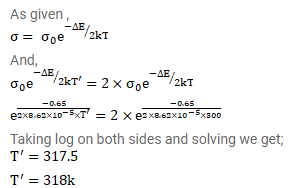# The conductivity of an intrinsic semiconductor depends on temperature as

Question:

The conductivity of an intrinsic semiconductor depends on temperature as $\sigma=\sigma_{0} \mathrm{e}^{-\Delta \mathrm{E} / 2 \mathrm{kT}}$ where $\sigma_{0}$ is a constant. Find the temperature at which the conductivity of an intrinsic germanium semiconductor will be double of its value at $\mathrm{T}=300 \mathrm{~K}$. Assume that the gap for germanium is $0.650 \mathrm{eV}$ and remains constant as the temperature is increased.

Solution: